GRE  >  Test: Coordinate Geometry- 1

# Test: Coordinate Geometry- 1

Test Description

## 15 Questions MCQ Test Quantitative for GMAT | Test: Coordinate Geometry- 1

Test: Coordinate Geometry- 1 for GRE 2023 is part of Quantitative for GMAT preparation. The Test: Coordinate Geometry- 1 questions and answers have been prepared according to the GRE exam syllabus.The Test: Coordinate Geometry- 1 MCQs are made for GRE 2023 Exam. Find important definitions, questions, notes, meanings, examples, exercises, MCQs and online tests for Test: Coordinate Geometry- 1 below.
Solutions of Test: Coordinate Geometry- 1 questions in English are available as part of our Quantitative for GMAT for GRE & Test: Coordinate Geometry- 1 solutions in Hindi for Quantitative for GMAT course. Download more important topics, notes, lectures and mock test series for GRE Exam by signing up for free. Attempt Test: Coordinate Geometry- 1 | 15 questions in 30 minutes | Mock test for GRE preparation | Free important questions MCQ to study Quantitative for GMAT for GRE Exam | Download free PDF with solutions
 1 Crore+ students have signed up on EduRev. Have you?
Test: Coordinate Geometry- 1 - Question 1

### If the x-intercept of line L is -5 and the slope is equal to 5/2, Find the y-intercept of the line L.

Test: Coordinate Geometry- 1 - Question 2

### Which of the following line is parallel to the given line 3x + 4y = 12 and has a negative y-intercept.

Test: Coordinate Geometry- 1 - Question 3

###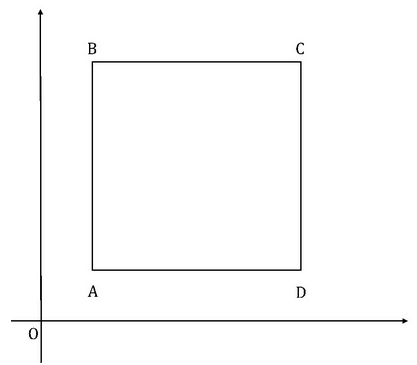In the given diagram, ABCD is a square and the coordinate of B is (5,5). If the length of each side is 2 units and AB is parallel to the y-axis, find the coordinate of D.

Test: Coordinate Geometry- 1 - Question 4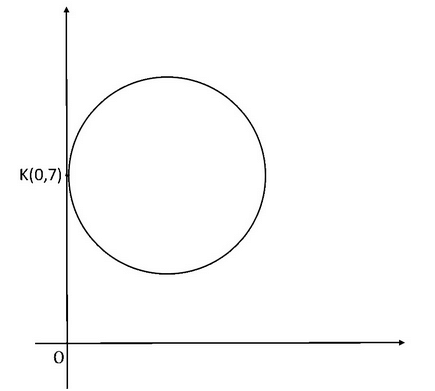In the given diagram, the circle touches the y-axis at the point K whose coordinate is (0,7). If the area of triangle CKO is 21 units2, where C is the centre of the circle, find the area of the circle.

Test: Coordinate Geometry- 1 - Question 5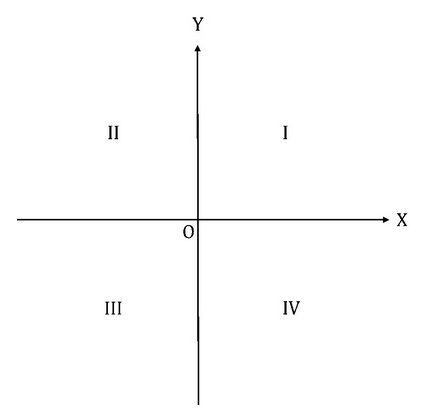The graph of the equation x2y = k, where k < 0, lies in which two quadrants shown above?

Test: Coordinate Geometry- 1 - Question 6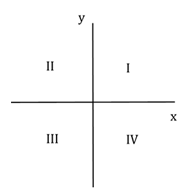Lines a and b, not shown, lie in the rectangular coordinate system shown above. Is the slope of line a greater than the slope of line b?

(1)   Line a does not intersect quadrant III.

(2)   Line b intersects quadrant IV.

Detailed Solution for Test: Coordinate Geometry- 1 - Question 6

Step 1 & 2 – Understand the question and draw inferences from the question statement.

Given: lines a and b are in the graph

To find: Comparison of the slopes of the lines

Step 3 – Analyze Statement 1 Independently

Per statement 1, line a does not intersect quadrant III

Inference 1: Line a can have a zero slope, a negative slope or an undefined slope. However, it cannot have a positive slope.

Since Statement 1 does not tell you anything about line b , the information provided in Statement 1 is not sufficient to arrive at a unique answer.

Step 4 – Analyze Statement 2 Independently

Per Statement 2, line b intersects quadrant IV

Inference 2: line b has any kind of slope

Inference 3: if the slope is positive, the y-intercept is negative

Since Statement 2 does not tell you anything about line a , the information provided in Statement 2 is not sufficient to arrive at a unique answer.

Step 5 – Analyze Both Statements Together

To analyze both statements together, we will put the inferences from both statements. Here is the data that we have:

Inference 1: Line a can't have a positive slope.

Inference 2: line b has any kind of slope

Inference 3: if the slope is positive, the y-intercept is negative

Though we know the slope of line a is negative, we know nothing of the slope of line b . Inference 3 does not help. The question cannot be answered.

Test: Coordinate Geometry- 1 - Question 7

A circle is tangent to the x-axis at 2 and the y-axis at 2. What is the area of the region between the circle and the origin?

Detailed Solution for Test: Coordinate Geometry- 1 - Question 7

Step 1: Question statement and Inferences

A circle tangent to the x-axis at 2 and the y-axis at 2 looks like this: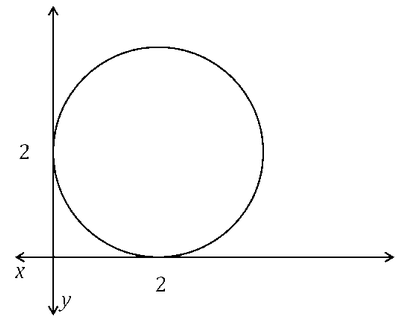You know that the radius of the circle is 2, making the area of the circle 4π.

Step 2: Finding required values

To find the region between the circle and the origin, measure the 2 × 2 square, and subtract the quarter circle from it. If the area of the circle is 4π, then the quarter circle is π.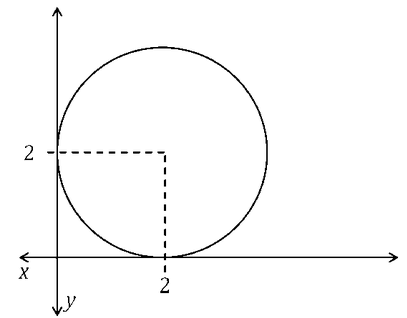Step 3: Calculating the final answer

4 – π is the area between the circle and the origin.

π  = 3.14 and 4 – 3.14 is less than 1.

Test: Coordinate Geometry- 1 - Question 8

If L1 passes through points in each quadrant except the IIIrd quadrant, then is the slope of the line L2 positive?

(1) L2 is perpendicular to L1.

(2) L1 and L2 intersect in the Ist quadrant.

Detailed Solution for Test: Coordinate Geometry- 1 - Question 8

Steps 1 & 2: Understand Question and Draw Inferences

If L1 passes through points in each quadrant except the IIIrd quadrant, then the Line must have both its x and y intercepts as positive numbers.

Let’s assume the x-intercept = a and y-intercept = b for the line L1, where both a and b are positive numbers. This means that the line L1 passes through (a, 0) and (0, b).

Slope of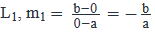. Since a and b are positive, the slope is a negative number.

Step 3: Analyze Statement 1

Slope of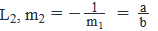mis positive since a and b are positive.

SUFFICIENT.

Step 4: Analyze Statement 2

If the slope of line L1 is negative and it intersects with a line L2 in the first quadrant, then the slope of L2 can be positive or negative as shown in the figures below: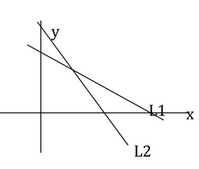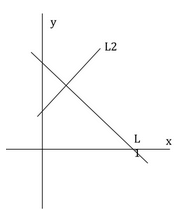The slope of Lin the first diagram is negative while in the second quadrant the slope of L2 is positive.

INSUFFICIENT.

Step 5: Analyze Both Statements Together (if needed)

Since we get an answer from Step 3, there is no need to combine the statements.

Test: Coordinate Geometry- 1 - Question 9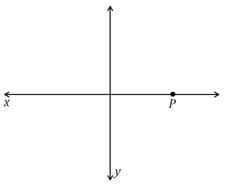On the x-y coordinate grid, if points P, Q, and R make a triangle (not shown), what is the area of the triangle?

(1) Point Q lies on the x-axis 6 units from point P.

(2) Point R is 5 units away from the x-axis

Detailed Solution for Test: Coordinate Geometry- 1 - Question 9

Steps 1 & 2: Understand Question and Draw Inferences

To find the area of the triangle, you need the base and the height.

With point P on the x-axis, knowing points Q and R will help you find the base and height.

Step 3: Analyze Statement 1

(1) Point Q lies on the x-axis 6 units from point P.

This gives you the base of the triangle. Whether point Q is left or right of point P, the triangle base is 6. However, you still need the height.

Statement 1 is not sufficient.

Step 4: Analyze Statement 2

(2) Point R is 5 units away from the x-axis

If the base of the triangle is on the x-axis, then knowing point R is 5 away gives you a height of 5. However, with this statement alone, you don’t have the base, and you don’t know whether the base is on the x-axis..

Statement 2 is not sufficient.

Step 5: Analyze Both Statements Together (if needed)

From statement 1, you know that the base is on the x-axis and that it has a length of 6.

From statement 2, because the base is on the x axis, you know the height is 5.

These are all you need to calculate the area of the triangle. The left-right placement of points Q and R don’t affect the area calculation:

Area = ½ * 6 * 5 = 15

Test: Coordinate Geometry- 1 - Question 10

On the x-y coordinate grid, are points A and B equidistant from the origin?

(1)       |x| = |y| for point A and |x| = |y| for point B

(2)       |x|, |y| of point A = |x|, |y| of point B

Detailed Solution for Test: Coordinate Geometry- 1 - Question 10

Steps 1 & 2: Understand Question and Draw Inferences

To find the distance from the origin, you need the absolute values of the (x, y) coordinates of points A and B.

To compare the distances of points A and B, you can use the ratios of the (x, y) values of these points.

Step 3: Analyze Statement 1

(1)       |x| = |y| for point A and |x| = |y| for point B

With statement 1, the |x|, |y| values of points A and B could be the same, or they could be different.

For instance, A could be (3, -3) and B could be (3, 3) or (4, -4).

Statement (1) is not sufficient.

Step 4: Analyze Statement 2

(2)       |x|, |y| of point A = |x|, |y| of point B

This tells you that points A and B are the same distance from the origin. You don’t know which quadrant the points are in, but you can still answer the question.

Statement (2) is sufficient.

Step 5: Analyze Both Statements Together (if needed)

You get a unique answer in step 4, so this step is not required

Test: Coordinate Geometry- 1 - Question 11

In which quadrant does the point (x,y) lie?

(1) (x, y+1) lies in the IInd Quadrant.

(2) (x + 3, y) lies in the IIIrd Quadrant.

Detailed Solution for Test: Coordinate Geometry- 1 - Question 11

Steps 1 & 2: Understand Question and Draw Inferences

We have to determine the specific quadrant in which the point (x, y) lies.

Step 3: Analyze Statement 1

Every point that lies in the IInd Quadrant has a negative x-coordinate and a positive y-coordinate. Since (x, y + 1) lies in the IInd Quadrant

x < 0    and     y + 1 > 0

x < 0    and     y > -1

Is x negative or positive? Negative.

Is y negative or positive? Since y is greater than -1, it can hold both negative and positive values.

If x is negative and y is negative then the point (x,y) lies in the IIIrd Quadrant.

If x is negative and y is positive then the point (x, y) lies in the IInd Quadrant.

INSUFFICIENT.

Step 4: Analyze Statement 2

Every point that lies in the IIIrd Quadrant has a negative x-coordinate and a negative y-coordinate. Since (x + 3, y) lies in the IIIrd Quadrant

x + 3 < 0  and  y < 0

x < -3       and   y < 0

Is x negative or positive? Since x is smaller than -3, it would always be a negative number.

Is y negative or positive? Negative.

Since x and y are both negative, the point (x, y) must lie in the IIIrd Quadrant.

SUFFICIENT.

Step 5: Analyze Both Statements Together (if needed)

Since we have obtained an answer, there is no need to combine the statements.

Test: Coordinate Geometry- 1 - Question 12

In the xy-plane, the line y = k is the perpendicular bisector of the line segment PQ and the line x = h is the perpendicular bisector of the line segment RQ. If the coordinates of the point R are (-h, -k), then what are the coordinates of the point P?

Detailed Solution for Test: Coordinate Geometry- 1 - Question 12

Step 1: Question statement and Inferences

Drawing the axes and the lines y = k and x = h given in the question on xy-plane: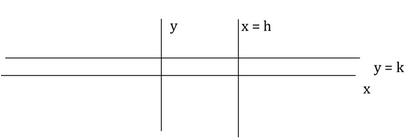Based on the figure drawn by us, plotting the point R whose coordinates are (-h, -k):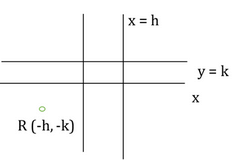Step 2:Finding required values

Since the line x = h is the perpendicular to QR, the y-coordinate of point R must also be equal to –k. RA and QA are perpendicular to the line x = h, the y-coordinate of R, A and Q are equal. Therefore, the coordinates of point A are (h, -k).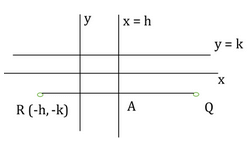RA = AQ = h – (-h) = 2h

The coordinates of point Q are (h + 2h, -k) or (3h, -k).

Step 3: Calculating the final answer

Since the line y = k is perpendicular to PQ, the x-coordinate of P, B and Q are equal, i.e. 3h.

Since QB = BP, and QB = k – (-k) = 2k, the y-coordinate of point P is k + 2k = 3k.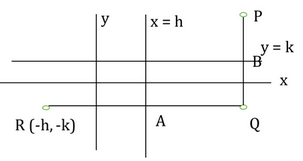The coordinates of the point P are (3h, 3k).

Test: Coordinate Geometry- 1 - Question 13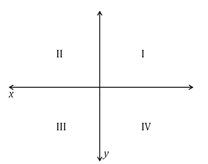On the x-y coordinate grid, does the line passing through point P (not shown) have a slope greater than 2?

(1) Point P has an x-value of 1 and is 2 units away from the origin

(2) The line does not pass through Quadrant IV

Detailed Solution for Test: Coordinate Geometry- 1 - Question 13

Steps 1 & 2: Understand Question and Draw Inferences

To find whether the slope is greater than 2, you need information on the orientation of the line. This can come from two points of the line or at least one point that the line passes above or below.

Step 3: Analyze Statement 1

(1) Point P has an x-value of 1 and is 2 units away from the origin

Statement 1 tells you that from the origin, point P creates a right triangle. Use the Pythagorean Theorem to find the y-value: (The triangle base is the x-value of 1, the hypotenuse is the distance of 2, and the height is the y-value)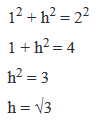The height of the triangle, which is the y-value of point p, has an absolute value of √3, making it either √3 or –√3. However, you need two points to draw the line, and you don’t know whether point P is in quadrant I or IV.

Statement 1 is not sufficient.

Step 4: Analyze Statement 2

(2) The line does not pass through Quadrant IV

Statement 2 tells you that the slope is positive (to avoid Quadrant IV), but you don’t know whether it’s greater than 2.

Statement 2 is not sufficient.

Step 5: Analyze Both Statements Together (if needed)

From statement 1, you know the (x, y) values of point P are either (1, √3) or (1, –√3), placing point P in either Quadrant I or IV.

From statement 2, you know that point P is in Quadrant I. You also know the line cannot cross into Quadrant IV.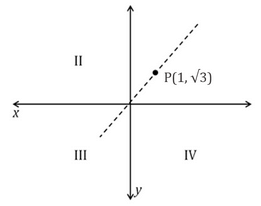As shown above, the slope is√3. The line can rotate clockwise, making the slope less, but it cannot rotate counterclockwise, as that would bring it into Quadrant IV. Taken together, you know that the slope is not greater than 2.

Statements 1 and 2 together are sufficient.

Test: Coordinate Geometry- 1 - Question 14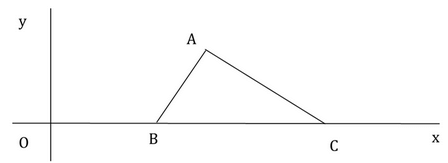In the rectangular coordinate system above, if OB < BC then is the area of the triangle ABC lesser than 12 square units?

(1) Point A lies above the line y = 5

(2) The mid-point of B and C is (6, 0)

Detailed Solution for Test: Coordinate Geometry- 1 - Question 14

Steps 1 & 2: Understand Question and Draw Inferences

In order to determine any information about the area of a triangle, we must try to obtain information about its base and height since

the area of a triangle =  (1/2)*base*height

If the base is taken as the length of line segment BC, then the height is the y-coordinate of point A.

Step 3: Analyze Statement 1

If the point A lies above the line y = 5 and the side BC lies on the x-axis, then the height of the triangle is more than 5 units. We have no information about the length of the base BC.

INSUFFICIENT.

Step 4: Analyze Statement 2

Let’s assume the coordinates of point B to be (b, 0) and the coordinates of point C to be (c, 0).

If the mid-point of B and C is (6, 0) then

(b+c)/2 = 6

c = 12 – b

Length of BC = 12 – b – b = 12 – 2b

Since OB < BC, we have

b < 12 – 2b

b < 4

We cannot infer anything about the base BC from this, apart from the fact that the length of BC will always be greater than 4 units.

There is also no information about the height of the triangle ABC.

INSUFFICIENT.

Step 5: Analyze Both Statements Together (if needed)

Combining statements (1) and (2),

We have Height > 5 and b < 4

We have information about the height but still we are unsure about the base BC and so we cannot conclude if the area of the triangle ABC is greater than 12 or less than 12.

INSUFFICIENT.

Test: Coordinate Geometry- 1 - Question 15

In the xy-plane, is the x-intercept of the line y = mx + b positive? (m and b are non-zero numbers)

(1) The y-intercept of the line m2y = bx + m is positive.

(2) The x-intercept of the line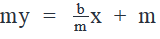is positive

Detailed Solution for Test: Coordinate Geometry- 1 - Question 15

Steps 1 & 2: Understand Question and Draw Inferences

Let’s find the x-intercept of the given line.

When the line intercepts the x-axis, the y-coordinate becomes 0.

Substituting y = 0 in the given equation of the line,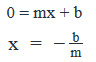The question thus becomes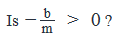Multiplying by -1 on both sides of the inequality, the question modifies to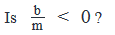Step 3: Analyze Statement 1

To compare the given line with the general form of the equation (y= mx + c), we divide both sides of the equation with m2.

The equation becomes y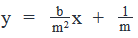we see that the y-intercept of the given line m2y = bx + m is 1/m

The statement tells us that 1/m>0

Using this information, we can only say that m > 0.

However, b/m can be negative or not depending on whether b is negative or not.

INSUFFICIENT.

Step 4: Analyze Statement 2

In order to find the x-intercept of the given equation of line my =  x + m, we substitute y = 0 in the given equation to get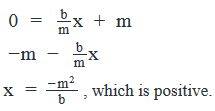Since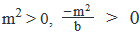only when b < 0.

Is b/m < 0 ?  We cannot be sure because we only know that b < 0. b/m

can be less than 0 or not depending on whether m is positive or not.

Step 5: Analyze Both Statements Together (if needed)

Combining statements (1) and (2),

we have m > 0 and b < 0.

This means that b/m < 0 for all such values of m and b.

SUFFICIENT.

## Quantitative for GMAT

86 videos|99 docs|165 tests
Information about Test: Coordinate Geometry- 1 Page
In this test you can find the Exam questions for Test: Coordinate Geometry- 1 solved & explained in the simplest way possible. Besides giving Questions and answers for Test: Coordinate Geometry- 1, EduRev gives you an ample number of Online tests for practice

## Quantitative for GMAT

86 videos|99 docs|165 tests(Scan QR code)